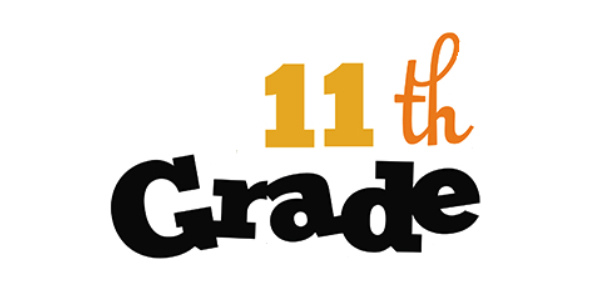# Capacitor Questions: 11th Grade Quiz!

15 Questions | Total Attempts: 694SettingsWhat do you know about capacitors? This quiz is for 11th graders. During this quiz, you will need to grasp how capacitors store energy, the parts of the capacitor, the difference between capacitor and battery, what the value of a capacitor depends on, and what the unit is of capacitance. You will also be presented pictures, and you must determine what they mean. You will truly benefit from taking this quiz.

• 1.
The capacitors stores energy in the form of:
• A.

Magnetic field

• B.

Electric field

• C.

Both magnetic and Electric field

• D.

Non of the above

• 2.
The parts of a Capacitor are:
• A.

Metal Plates and Dielectric

• B.

Metal Plates and Charges

• C.

One Dielectric and two metal plates

• D.

All of the above

• 3.
The differences between Capacitor and Battery is:
• A.

Shape

• B.

No chemical is used

• C.

Length

• D.

Size

• 4.
The unit of capacitance is:
• A.

• B.

• C.

• D.

• 5.
The picture shows the symbol of:
• A.

Polarized Capacitor

• B.

Variable Capacitor

• C.

Non Polarized Capacitor

• D.

Non of the above

• 6.
The picture shows the symbol of
• A.

Non polarized capacitor

• B.

Polarized capacitor

• C.

Variable capacitor

• D.

All of the above

• 7.
The picture shows the symbol of
• A.

Fixed Capacitor

• B.

Variable Capacitor

• C.

Series Capacitor

• D.

Non Variable Capacitor

• 8.
A capacitor is connected with battery, What will happen to the capacitor?
• A.

Capacitor will get damage

• B.

Capacitor will not damage

• C.

Capacitor may damage

• D.

All of the above

• 9.
The value of Capacitor depend on:
• A.

Plate area, Distance between plate and dielectric

• B.

Only on Plate area, Distance between plate

• C.

Only on Distance between plate and dielectric

• D.

All of the above

• 10.
The formula is used to calculate the
• A.

Capacitance

• B.

Resistance

• C.

Capacitive reactance

• D.

Frequency of applied voltage

• 11.
The picture shows capacitors are connected in
• A.

Parallel

• B.

Parallel as well as series

• C.

Series

• D.

Non of the above

• 12.
The formula is used to calculate the total capacitance in
• A.

Parallel

• B.

Series

• C.

Can be used for both

• D.

Non of the above

• 13.
The formula is used to calculate the total capacitance in
• A.

Parallel

• B.

Series

• C.

Can used for both

• D.

All of the above

• 14.
• A.

O.1 F

• B.

0.001 F

• C.

0.00001 F

• D.

0.0000001 F

• 15.
The total capacitance is
• A.

• B.Back to top